# Precalculus : Matrices and Vectors

## Example Questions

### Example Question #1 : Find The Multiplicative Inverse Of A Matrix

What is the inverse of the following nxn matrix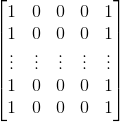The matrix is not invertible.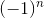The matrix is not invertible.

Explanation:

Note the first and the last columns are equal.

Therefore, when we try to find the determinant using the following formula we get the determinant equaling 0: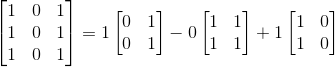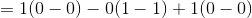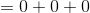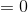This means simply, that the matrix does not have an inverse.

### Example Question #1 : Find The Multiplicative Inverse Of A Matrix

Find the inverse of the matrix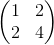.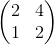Does not exist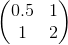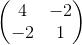Does not exist

Explanation:

For a 2x2 matrix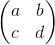the inverse can be found by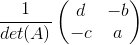Because the determinant is equal to zero in this problem, or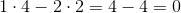,

the inverse does not exist.

### Example Question #3 : Inverses Of Matrices

Find the inverse of the matrix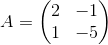.None of the other answers.Explanation:

There are a couple of ways to do this. I will use the determinant method.

First we need to find the determinant of this matrix, which is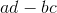for a matrix in the form:.

Substituting in our values we find the determinant to be: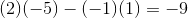Now one formula for finding the inverse of the matrix is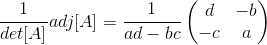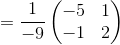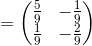.

### Example Question #4 : Inverses Of Matrices

Find the inverse of the matrix.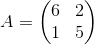Explanation:

We use the inverse of a 2x2 matrix formula to determine the answer. Given a matrix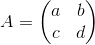it's inverse is given by the formula: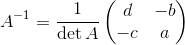First we define the determinant of our matrix: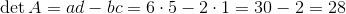Then,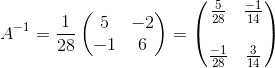### Example Question #5 : Inverses Of Matrices

Find the inverse of the following matrix.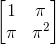This matrix has no inverse.This matrix has no inverse.

Explanation:

This matrix has no inverse because the columns are not linearly independent. This means if you row reduce to try to compute the inverse, one of the rows will have only zeros, which means there is no inverse.

### Example Question #1 : Find The Multiplicative Inverse Of A Matrix

Find the multiplicative inverse of the following matrix: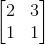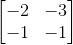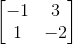This matrix has no inverse.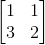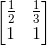Explanation:

By writing the augmented matrix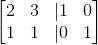, and reducing the left side to the identity matrix, we can implement the same operations onto the right side, and we arrive at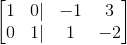, with the right side representing the inverse of the original matrix.

### Example Question #7 : Inverses Of Matrices

What is the inverse of the identiy matrix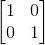?

The identity matrixThe identity matrixExplanation:

By definition, an inverse matrix is the matrix B that you would need to multiply matrix A by to get the identity. Since the identity matrix yields whatever matrix it is being multiplied by, the answer is the identity itself.

### Example Question #1 : Find The Sum And Difference Of Vectors

Evaluate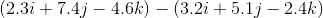None of the other answersExplanation:

When adding two vectors, they need to be expanded into their components. Luckily, the problem statement gives us the vectors already in their component form. From here, we just need to remember that we can only add like components. So for this problem we get: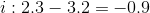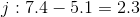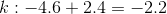Now we can combine those values to write out the complete vector: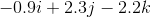### Example Question #1 : Find The Sum And Difference Of Vectors

What is the magnitude and angle for the following vector, measured CCW from the x-axis?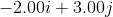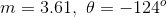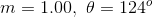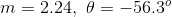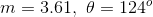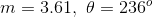Explanation:

The magnitude of the vector is found using the distance formula: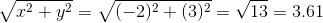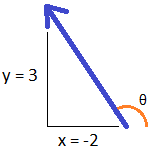To calculate the angle we must first find the inverse tangent of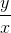: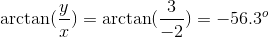This angle value is the principal arctan, but it is in the fourth quadrant while our vector is in the second. We must add the angle 180° to this value to arrive at our final answer.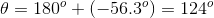### Example Question #1 : Find The Sum And Difference Of Vectors

Vector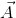has a magnitude of 3.61 and a direction 124° CCW from the x-axis. Express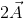in unit vector form.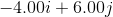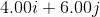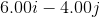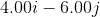Explanation:

For vector, the magnitude is doubled, but the direction remains the same.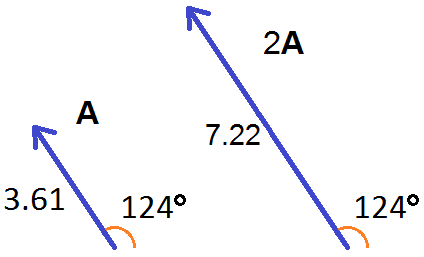For our calculation, we use a magnitude of: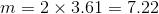The x-coordinate is the magnitude times the cosine of the angle, while the y-coordinate is the magnitude times the sine of the angle.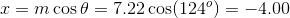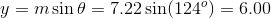The resultant vector is:.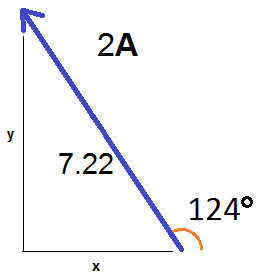### All Precalculus Resources